Formation and order of matrix

Chapter 3 Class 12 Matrices
Concept wise

For matrix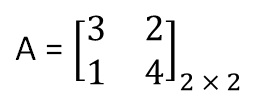Number of elements = 2 × 2 = 4

We can calculate number of elements by counting,

or from order of matrix

Number of elements = Number of Rows × Number of columns

Similarly, for matrices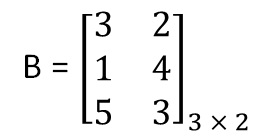Number of elements = 3 × 2

= 6

For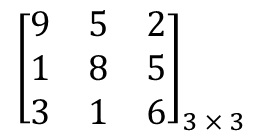Number of elements = 3 × 3 = 9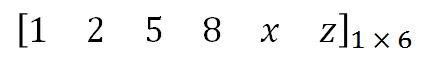Number of elements = 1 × 6 = 6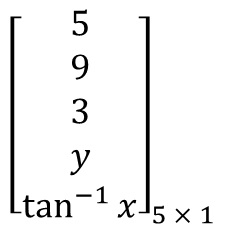Number of elements = 5 × 1 = 5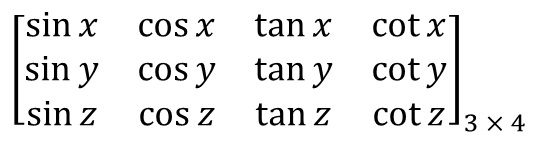Number of elements = 3 × 4 = 12

## If a matrix has 12 elements, what are the possible order it can have?

Since matrix has 12 elements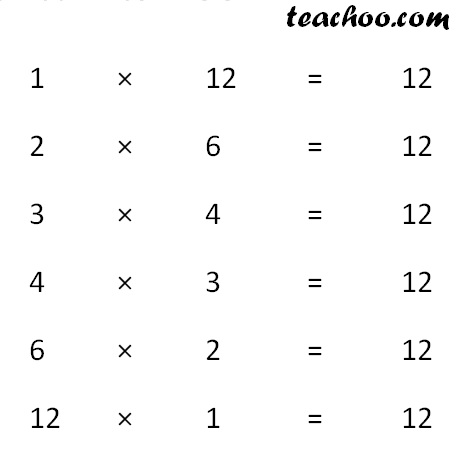Hence, possible order are

1 × 12, 2 × 6, 3 × 4, 4 × 3, 6 × 2, 12 × 1

Learn in your speed, with individual attention - Teachoo Maths 1-on-1 Class

### Transcript

A = [■8(3&2@1&4)]_(2 × 2) B = [■8(3&2@1&4@5&3)]_(3 × 2) [■8(9&5&2@1&8&5@3&1&6)]_(3 × 3) [■8(1&2&5&8&𝑥&𝑧)]_(1 × 6) [■8(5@9@3@𝑦@tan^(−1)⁡𝑥 )]_(5 × 1) [■8(sin⁡𝑥&cos⁡𝑥&tan⁡𝑥&cot⁡𝑥@sin⁡𝑦&cos⁡𝑦&tan⁡𝑦&cot⁡𝑦@sin⁡𝑧&cos⁡𝑧&tan⁡𝑧&cot⁡𝑧 )]_(3 × 4)